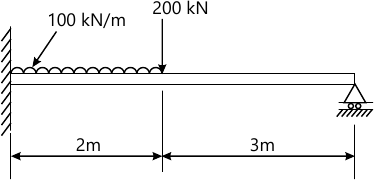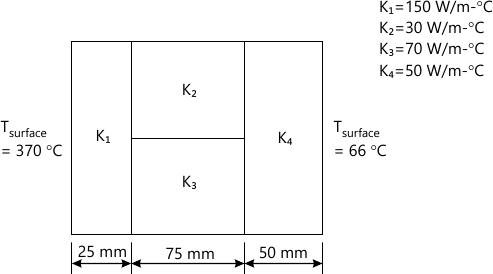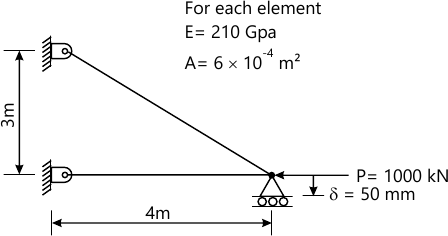MORE IN Finite Element Analysis
MU Mechanical Engineering (Semester 8)
Finite Element Analysis
December 2013
Total marks: --
Total time: --
INSTRUCTIONS
(1) Assume appropriate data and state your reasons
(2) Marks are given to the right of every question
(3) Draw neat diagrams wherever necessary

1(a) Derive transformation matrix for a plane truss element,relating displacement in the local(element)coordinates and global coordinates.
5 M
1(b) Explain different types of boundary condition giving examples.
5 M
1(c) What is the difference between shape function and interpolation function?Derive and sketch the same for linear bar element.
5 M
1(d) What do you mean by consistent and lumped mass matrices?Derive the same for linear bar element.
5 M

2(a) Solve the following differential equation by:
Collocation method
5 M
2(b) Galerkin method.
5 M
2(c) Rayleigh ritz method mapped over entire domain
$\dfrac{d^{2}u}{dx^{2}}+u=x^{2}\$ 0&leq ;x ≤ 1
BcS; u(0)=0 $\dfrac{du}{dx}|_{x=1}=1\$
Compare the answer with exact solution at x =0.25,0.5,0.75 and 1.
10 M

3(a) The following differential equation arises in connection with heat transfer in an insulated rod:
$\dfrac{d}{dx} \left(-k\dfrac{dT}{dx}\right)=q$ for 0≤x≤L
BcS;T(0)=To\left[k\dfrac{dT}{dx}+?(T-T_{&infty;})+q\right]_{x=L}=0\]
Where T is the temperature, K is the thermal conductivity and q the heat generation.Take the following values for the data;
L=0.1m,K=0.01 w/m -°C,β=25 W/m-°C,q=q=0
To =50° and T=5°C. Solve the problem using two linear finite elements for temperature values at x =L/2 and X=L. Derive the equation which you use.
14 M
3(b) Explain the principle of minimum total potential with suitable example.
6 M

4(a) Find the deflection and slopes at nodes and reaction at support for the beam as shown in figure below.Take EI=4000KN-m210 M
4(b) Find the heat transfer per unit area through the composite wall as shown in figure below.10 M

5(a) What do you understand by Jacobin matrix? Derive the same for four-noded quadrilateral element.
15 M
5(b) The triangular element has nodes coordinates (10,10),(40,20)and (30,50) for nodes 1,2,and 3 respectively.For the point P located inside the triangle,determine the x and y coordinates if the shape function N1 and N2 are 0.15 and 0.25 respectively.
5 M

6(a) Analyse the following truss completely,i.e for displacement,reaction and stressesThe nodes where load is applied settles by an amount &delta =50mm as shown in figure.
14 M
6(b) Write any one algorithm for the solution of Eigen values problem.
6 M

7(a) Evaluate the following integral by Newton-cotes and Gauss-quadrature formula.
$I =\int_{0}^{le}\dfrac{dN1}{dx}0\frac{dN2}{dx}\bar{dx}\$
Where,$N1 =\left(1-\dfrac{\bar{x}}{le}\right)\left(1-\dfrac{\bar{2x}}{le}\right)\$
$N_{2}=\dfrac{\bar{4x}}{le}\left(1-\dfrac{\bar{x}}{le}\right)\$,br>Check your answer with exact value.
Use following table
For Newton-cotes.
 No.of sampling points W1 W2 W3 W4 1 1 - - - 2 1/2 1/2 - - 3 1/6 4/6 1/6 - 4 1/8 3/8 3/8 1/8

 No.of sampling points ? i Wi 1 0 2 2 $\pm \dfrac{1}{\sqrt{3}} 1 3 \[\dfrac{0}{\pm\sqrt{0.6}}\$ 8/9 5/9